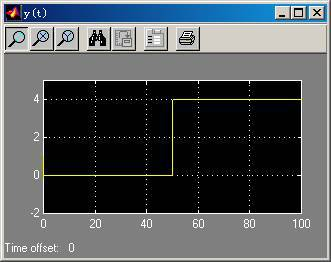More PID Loops Tuned by BESTune

Shown below is the PID control system designed using Simulink. The reference signal (setpoint) changes from 0 to 4 at time t=50. The PID controller is tuned using the BESTune technology. This simulation program can be downloaded by RIGHT (not left) clicking on this link and choose "save as...".(You need Matlab and Simulink of MathWorks Inc to run this simulation).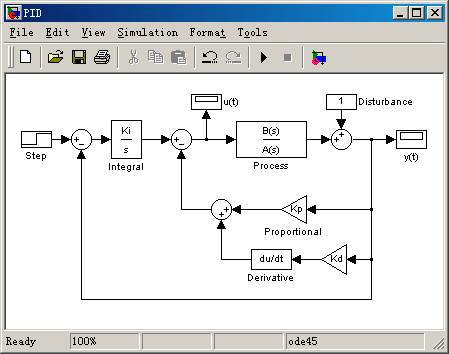1. A=(s+1)8, B=1 (Used by Eurotherm and Foxboro to test PID controller performance): The performance is shown below.

(1)   The optimal tuning is done for PID controller: Kp=0.3515, Ki=0.0911, and Kd=0.3516.

(2)   The optimal tuning is done for PI controller: Kp= 0.1722, Ki=0.0680, and Kd=0.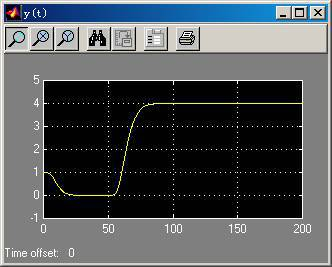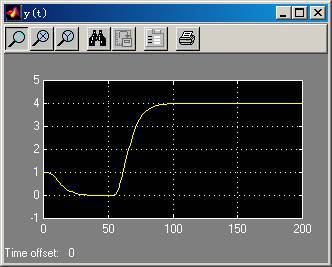1. A=(s+1)(0.1s+1)(0.01s+1)( 0.001s +1), B=1. The controller performance is shown below.

(1)   The optimal tuning is done for PID controller: Kp=77.2731, Ki=522.2601, and Kd=3.3083 (Left figure).

(2)   The optimal tuning is done for PI controller: Kp=2.8479, Ki=4.4986, Kd=0 (Right figure).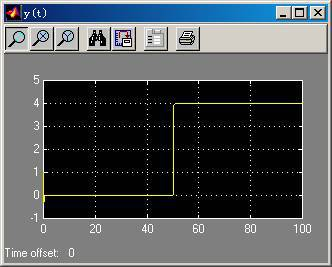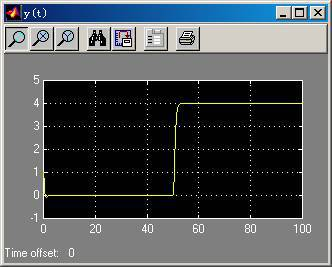1. A=(s+1)3, B=1-5s. The performance is shown below.

(1)   The optimal tuning is done for PID controller: Kp= 0.2440, Ki=0.1032, and Kd=0.1467 (Left figure).

(2)   The optimal tuning is done for PI controller: Kp= 0.1251, Ki=0.0787, and Kd=0 (Right figure).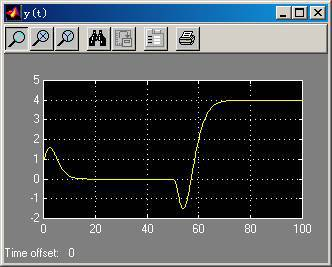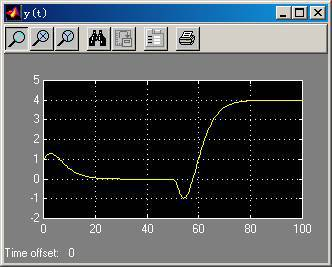1. A=(s+1)(s2+0.2s+1), B=1. The performance is shown below.

(1)   The optimal tuning is done for PID controller: Kp= 0.9947, Ki=0.3155, Kd=2.4846 (Left figure).

(2)   The optimal tuning is done for I-only controller: Kp= 0, Ki=0.0743, and Kd=0 (Right figure).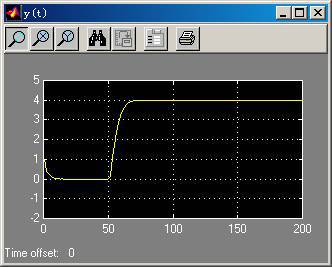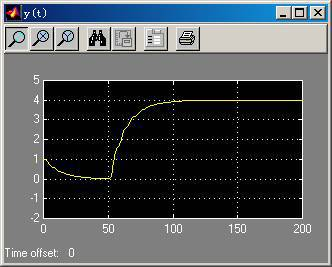1. A=s2-1, B=1. The performance is shown below.

(1)   The optimal tuning is done for PID controller: Kp= 1393.4, Ki=9998.8, Kd=64.6 (Left figure).

(2)   The optimal tuning is done for PID controller with the constraints that the parameters are not allowed to be larger than 9: Kp= 9.0000, Ki=4.3547, and Kd=4.8989 (Right figure).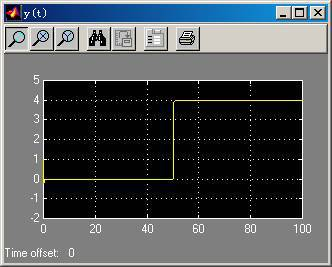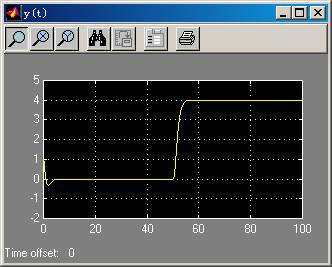1. A=s, B=1. The performance is shown below.

(1)   The optimal tuning is done for PI controller with the constraints that the parameters are not allowed to be larger than 9999: Kp= 200.0, Ki=9999, Kd=0 (Left figure).

(2)   The optimal tuning is done for PI controller with the constraints that the parameters are not allowed to be larger than 5: Kp=4.4721, Ki=5, and Kd=0 (Right figure).